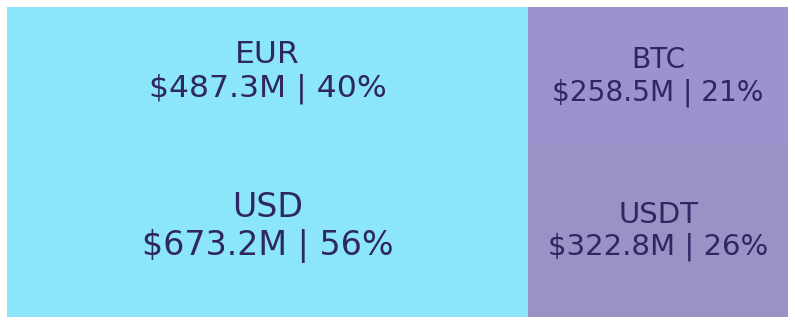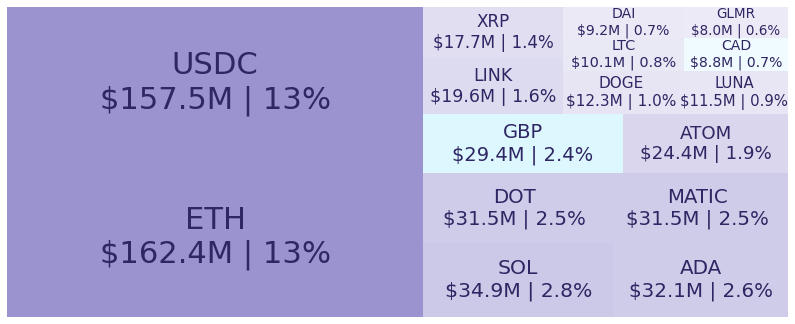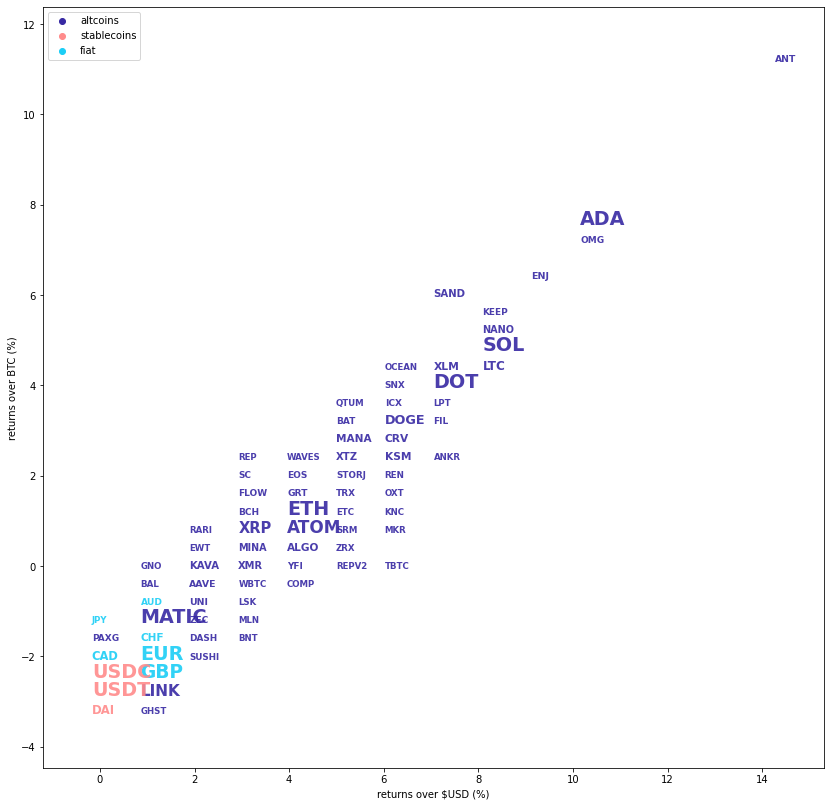# Kraken Daily Market Report for January 12 2022

## Overview

• Total spot trading volume at $1.23 billion, the 30 day average is$1.14 billion.
• Total futures notional at $225.2 million. • The top traded coins were, respectively, Tether (0%), Bitcoin (+2.8%), Ethereum (+4.1%), USDC (+0%), and Solana (+8.1%). • Aragon (+14%) has had an interesting 30 days: lows near$4.2 and highs near $14.0. Aragon closed today at$9.45.

January 12, 2022
$1.23B traded across all markets today Crypto, EUR, USD, JPY, CAD, GBP, CHF, AUD USDT$1.0004
↑0.0%
$322.8M BTC$43927.
↑2.8%
$258.5M ETH$3373.7
↑4.1%
$162.4M USDC$1.0001
↑0.01%
$157.5M SOL$151.56
↑8.1%
$34.9M ADA$1.3128
↑11%
$32.1M DOT$27.317
↑6.8%
$31.5M MATIC$2.3964
↑1.3%
$31.5M ATOM$40.225
↑4.1%
$24.4M LINK$26.759
↑0.6%
$19.6M XRP$0.7980
↑3.5%
$17.7M DOGE$0.1629
↑6.0%
$12.3M LUNA$81.934
↑11%
$11.5M LTC$141.91
↑7.8%
$10.1M DAI$1.0000
↓0.0%
$9.17M GLMR$8.987
↓24%
$8.01M XLM$0.2823
↑7.2%
$6.42M CRV$4.8984
↑6.0%
$6.33M KSM$275.54
↑5.7%
$6.2M AVAX$95.95
↑7.1%
$5.87M MANA$3.0896
↑5.2%
$5.76M ALGO$1.5012
↑3.8%
$5.76M KAVA$4.9695
↑2.2%
$4.67M SAND$5.1396
↑7.4%
$4.66M XTZ$4.3843
↑5.0%
$4.19M XMR$199.24
↑3.2%
$3.87M NANO$3.2877
↑7.8%
$3.7M SHIB$3.15e-
↑14%
$3.5M MINA$3.59
↑3.5%
$3.2M ENJ$2.521
↑8.9%
$2.8M AAVE$218.62
↑2.3%
$2.62M UNI$16.267
↑1.7%
$2.34M LRC$1.6281
↑8.1%
$2.21M ANT$9.4513
↑14%
$2.13M GRT$0.5945
↑4.3%
$1.84M ZEC$144.42
↑1.9%
$1.59M MOVR$169.99
↑2.7%
$1.58M EOS$2.8842
↑3.9%
$1.55M BCH$383.81
↑3.7%
$1.53M PAXG$1832.4
↑0.3%
$1.52M FLOW$7.936
↑3.8%
$1.42M BAT$1.1158
↑5.4%
$1.41M ICX$1.0427
↑5.8%
$1.36M OMG$5.895
↑11%
$1.33M FIL$30.747
↑6.4%
$1.26M TRX$0.0681
↑4.9%
$1.22M YFI$33811.
↑3.3%
$1.16M SC$0.0140
↑3.4%
$1.11M CTSI$0.6873
↑18%
$1.1M SNX$5.425
↑6.2%
$1.04M DYDX$7.779
↑8.9%
$946K KEEP$0.6681
↑8.0%
$932K DASH$143.52
↑1.3%
$892K CHZ$0.2778
↑4.8%
$771K REN$0.488
↑5.5%
$659K SUSHI$7.13
↑1.6%
$627K AXS$78.191
↑7.5%
$584K BAL$17.92
↑1.5%
$547K STORJ$1.6670
↑4.7%
$545K OCEAN$0.7897
↑5.7%
$508K ETC$31.156
↑4.9%
$502K QTUM$7.9175
↑5.1%
$453K KILT$3.3566
↑14%
$429K OXT$0.3264
↑5.3%
$413K LPT$39.28
↑6.2%
$378K LSK$2.1487
↑2.1%
$360K INJ$7.224
↑0.3%
$357K WAVES$14.775
↑4.5%
$353K SRM$3.175
↑4.3%
$336K WBTC$43982.
↑2.6%
$335K BAND$5.659
↑7.0%
$332K KNC$1.4011
↑4.8%
$314K EWT$8.452
↑2.1%
$313K REP$17.034
↑3.9%
$306K COMP$196.79
↑3.6%
$302K KINT$31.73
↓6.2%
$298K RARI$13.62
↑2.1%
$263K MLN$77.342
↑2.4%
$261K GNO$408.69
↑1.8%
$193K PHA$0.379
↑4.1%
$187K KAR$3.457
↑3.2%
$185K PERP$9.514
↑0.8%
$161K CQT$0.5080
↑1.8%
$147K 1INCH$2.246
↑4.4%
$140K ANKR$0.0975
↑6.9%
$130K BNC$1.57
↑0.0%
$115K SDN$1.501
↓0.3%
$97.4K BADGER$12.709
↑4.7%
$94.4K GHST$2.1768
↑1.0%
$90.1K MKR$2176.4
↑5.4%
$90.1K RAY$5.541
↑4.2%
$89.2K OXY$0.5859
↑7.5%
$80.4K MIR$1.8090
↑2.0%
$71.7K REPV2$17.08
↑4.7%
$67.7K ZRX$0.753
↑4.9%
$60.4K OGN$0.5398
↑4.0%
$43.5K BNT$3.2739
↑2.2%
$32.5K TBTC$46456.
↑6.2%
\$11.5K

#####################. Trading Volume by Asset. ##########################################

The figures below break down the trading volume of the largest, mid-size, and smallest assets. Cryptos are in purple, fiats are in blue. For each asset, the chart contains the daily trading volume in USD, and the percentage of the total trading volume. The percentages for fiats and cryptos are treated separately, so that they both add up to 100%.

Figure 1: Largest trading assets: trading volume (measured in USD) and its percentage of the total trading volume (January 12 2022)Figure 2: Mid-size trading assets: (measured in USD) (January 12 2022)###########. Daily Returns. #################################################

## Daily Returns %

Figure 3: Returns over USD and XBT. Relative volume and return size is indicated by the size of the font. (January 12 2022)###########. Disclaimer #################################################

The values generated in this report are from public market data distributed from Kraken WebSockets api. The total volumes and returns are calculated over the reporting day using UTC time.